Journal of Electrical and Electronic Engineering
Volume 3, Issue 3, June 2015, Pages: 36-41

Effects of Distributed Generation on System Power Losses and Voltage Profiles (Belin Distribution System)

Chaw Su Hlaing, Pyone Lai Swe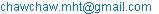(C. S. Hlaing)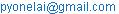(P. L. Swe)

Chaw Su Hlaing, Pyone Lai Swe. Effects of Distributed Generation on System Power Losses and Voltage Profiles (Belin Distribution System). Journal of Electrical and Electronic Engineering. Vol. 3, No. 3, 2015, pp. 36-41. doi: 10.11648/j.jeee.20150303.13

Abstract: In present times, the use of DG systems in large amounts in different power distribution systems has become very popular and is growing on with fast speed. Although it is considered that DG reduces losses and improves system voltage profile, this paper shows that this is usually true. The paper presents voltage stability index based approach which utilizes combine sensitivity factor analogy to optimally locate and size a multi-type DG in 48-bus Belin distribution test system with the aim of reducing power losses and improving the voltage profile. The multi-type DG can operate as; type 1 DG (DG generating real power only), and type 2 DG (DG generating both real and reactive power). It further shows that the system losses are reduced and the voltage profile improved with the location of type 2 DG than with the location of type 1 DG. It reaches a point where any further increase in number of DGs in the network results for minimizing power losses and voltage profiles improvement.

Keywords: Distributed generation (DG), Voltage stability index (SI), System Loss Reduction, Voltage Profiles Improvement, Optimal Locating and Sizing

1. Introduction

Distributed generation (DG) is small-scale power generation that is usually connected to distribution system. The Electric Power Research Institute (EPRI) defines DG as generation from a few kilowatts up to 50MW . Ackermann et al. have given the most recent definition of DG as: "DG is an electric power generation source connected directly to the distribution network or on the customer side of the meter." .

In most power systems, a large portion of electricity demand is supplied by large-scale generators. This is because of economic advantages of these units over small ones. The distributed real power sources can be classified into two categories and referred in the following sections of this paper as type 1 DG and type 2 DG:

Type 1 DG: Distributed generations that supply real power, depending on the availability or demand, to the network without demanding any reactive power. Few examples of type 1 DG are photovoltaic cell, fuel cell, battery storage.

Type 2 DG: Distributed generations that supply both active and reactive power to the network. Type 2 is used for DG sources such as wind generation, combustion engines, and like synchronous generators .

Normally, the real power loss reduction draws more attention for the utilities, as it reduces the efficiency of transmitting energy to customers. Nevertheless, reactive power loss is obviously not less important. This is due to the fact that reactive power flow in the system needs to be maintained at a certain amount for sufficient voltage level. Consequently, reactive power makes it possible to transfer real power through transmission and distribution lines to customers . System loss reduction by strategically placed DG along the network feeder can be very useful if the decision maker is committed to reduce losses and to improve network performance maintaining investments to a reasonable low level .

Studies indicate that poor selection of location and size of a DG in a distribution system would lead to higher losses than the losses without DG. In a power system, the system operator is obligated to maintain voltage level of each customer bus within the required limit . Actually in practice, many electricity companies try to control voltage variations within the range of ±5% . The DG units improve voltage profiles by changing power flow patterns. The locations and size of DGs would have a significant impact on the effect of voltage profile enhancement.

2. Voltage Stability Index

A system experiences a state of voltage instability when there is a progressive or uncontrollable drop in voltage magnitude following a disturbance, increase in load demand or change in operating condition. It is usually identified by an index called steady state voltage stability index, evaluated using sensitivity analysis. Sensitivity analysis is the computation of voltage stability index of all the nodes in RDS. Voltage stability index, SI can be computed as follows:

SI Index, proposed by , is utilized to find the weakest voltage bus in power system. This index will find the most optimum weakest link in the system which could lead to voltage stability in future, when the load will increase. The value of index is given by Eq. (1) and termed as Stability index (SI).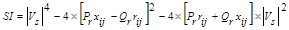(1)

where, SI is the stability index, Vs is the sending bus voltage, Pr is active load at receiving end, Qr is the reactive load at receiving end, rij is the resistance of the line i-j and xij is reactance of the line i-j.

Under stable operation, the value of SI should be greater than zero for all buses. When the value of SI becomes closer to one, all buses become more stable. In the proposed algorithm, SI value is calculated for each bus in the network and sort from highest to lowest value. For the bus having the lowest value of SI, will be considered in fitness function.

3. Objective Function

As the main objective of this work is to determine the optimal location and sizing of the distributed generation in the distribution network to minimize the losses (active power loss), the following objective function is selected as :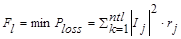(2)

where, Fl is the objective function to minimize power losses. Ploss is the active power loss. ntl is the number of lines in the distribution system.

Subjected to constraints: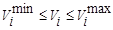(3)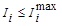(4)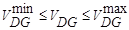(5)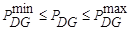(6)

where,

PDG =real power generations of DG

Vi =voltage magnitudes at bus i

VDG =voltage magnitudes at bus i

4. Problem Formulation

The problem formulation for the optimal location and sizing of the distributed generation in the distribution network to minimize the active power loss includes the power flow with and without distributed generation in the distribution system. The distributed generation is considered as active power sources at a particular voltage, which is at unity power factor. The well-known basis load flow equations are :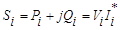(7)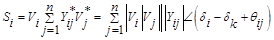(8)

Resolving into the real and imaginary parts, then the power flow equations without DG are given as: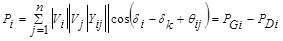(9)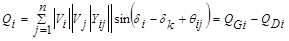(10)

The basic power balance equations: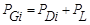(11)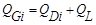(12)

The power flow equations considering losses with DG for the practical distribution system and the DG is an active power source at unity power factor (PV generator) then flow are given as: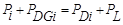(13)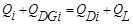(14)

The DG is active power source only at unity power factor, so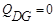.(15)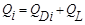(16)

The final power flow equations for distribution system are: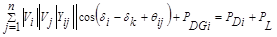(17)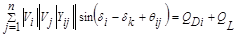(18)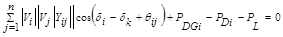(19)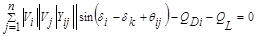(20)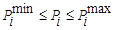(21)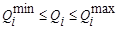(22)(23)(24)

where,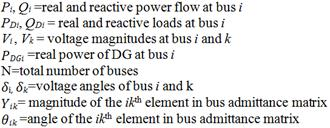5. Solution Methodology

Following steps are involved in optimal siting and sizing of distributed generations:

Step: 1Determination of proposed locations for placing distributed generations

a) Perform load flow analysis to calculate the bus voltage magnitudes and total network power loss in the RDS.

b) Compute the voltage stability index (SI) using Eq. (1).

c)  Arrange the buses in ascending order of the voltage stability index and select one or two buses with low value of voltage stability index from different laterals as the proposed locations for placing distributed sources.

Step: 2 Run the Base Case without DG using NR load flow using MATLAB software and calculate the bus voltage magnitude, angle, and real and reactive power loss respectively.

a) After Load flow identify the optimum sizing for each bus is calculated.

b) Find out the approximate losses for each bus by placing DG at the corresponding location with the optimum sizing obtains from the above step.

c)  Check for constraint violation after DG placement.

d) Locate the bus at which the loss is minimum after DG placement and this is the optimum location for DG.

Repeat the above procedure till the termination condition is satisfied.

6. Results and Discussions

The solution methodology presented in this paper for optimal siting and sizing of distributed power sources are analyzed using 132 kV, 33 kV and 11 kV, 48-bus Belin distribution system. The data for 48-bus Belin distribution system is given in Table 4 in the appendix.

This system is supplied from Yeywa Generation Station of 487 MW and 400 MVAR, 230 kV with a total peak load of 79.53 MW and 41.87 MVAR. The total system power loss at the peak demand without DG connection (base case scenario) is 1.351MW and 17.56 MVAR. The single line diagram of 48-bus Belin distribution system is given in Fig.1.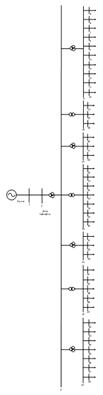Figure 1. The 48-bus Belin distribution system.

6.1. Optimum Size Allocation

The number of DGs to be included in a power network can be limited by several factors. The two main factors are the undesirable effects on power system parameters and the economic factors. This research was mainly concerned with the system power losses and the voltage profile of the network and thus the effects of DG on these system parameters have been investigated. The DG limits were taken to be as follows; 0 MW – 53 MW for real power limit (Type 1, and 2 DGs), 0 MVAR – 31 MVAR for reactive power limit (Type 2 DG).

After calculating the combined sensitivity factors, the buses were arranged in order of sensitivity and those with a factor of less than 0.8 were selected as the proposed buses. Table 1 shows the results of the optimal DG sizes for each respective proposed location and the associated best fitness achieved for all the two types of DGs. Both real and reactive power losses are considered in while investigating the effect of DG on system power losses. The number of DGs was assumed to increase from one, two, three and then four. This was done sequentially ensuring that the proposed bus with the most optimal size was chosen first followed with the others in the same order. Thus the most optimal DG location and size was included in the four cases.

Table 1. Results for SI and optimal DG sizes for multi-type DGs located on chosen proposed buses.

 Proposed Bus SI index Type 1 DG Type 2 DG Optimal DG Size (MW) Optimal DG size (MW+jMVAR) 10 0.7827 6.4635 10.5250+j5.5639 24 0.6577 6.0899 12.9831+j5.9928 25 0.7082 6.1199 10.0045+j5.9844 26 0.7750 9.7073 52.3274+j30.8305 27 0.7349 6.7413 10.3567+j5.4865 28 0.7688 3.4966 7.4777+j3.9912 29 0.7663 6.5099 9.0574+j4.8620 30 0.7607 5.2389 3.4092+j1.8000 36 0.7781 4.6756 17.6717+j9.3471 37 0.7813 1.8338 6.4406+j3.4067 38 0.7118 4.8256 4.9556+j2.6225 39 0.7613 2.5351 1.9437+j1.0251 40 0.7884 2.5446 1.0499+j0.5530 43 0.7953 2.8892 6.2551+j3.3038 46 0.7794 3.4975 2.3135+j1.2223

Table 2. Effects of type 1 DG on system power losses.

 Number of DGs Bus No. DG Size Power Losses % Power Loss Reduction MW MW+jMVAR %(MW+jMVAR) One 24 6.0899 1.125+j15.16 16.73+j13.67 Two 24 6.0899 0.971+j13.19 28.13+j24.89 25 6.1199 Three 24 6.0899 0.887+j11.91 34.34+j32.18 25 6.1199 38 4.8256 Four 24 6.0899 0.774+j10.18 42.71+j42.03 25 6.1199 38 4.8256 27 6.7413

As it can be seen from Table 2, the introduction of only one type 1 DG on bus 24 reduced the real power losses from the base case scenario of 1.351 MW to 1.125 MW and the reactive losses from 17.56 MVAR to 15.16 MVAR. The inclusion of the second DG in the system further reduced both real and reactive power losses to 0.971 MW and 13.19 MVAR. The introduction of the third DG reduces both real and reactive power losses to 0.887 MW and 11.91 MVAR. The inclusion of the fourth DG in the system results to 0.774 MW and 10.18 MVAR less than the case without DG in both real and reactive power losses.

From the results in Table 3, the introduction of the first optimally placed and sized type 2 DG in the network reduced the real power losses from the base case value of 1.351 MW to 1.135 MW and the reactive power losses from 17.56 MVAR to 12.45 MVAR. The inclusion of the second and third DG in the network further reduces the real power losses to 1.019 MW and 0.915 MW and the reactive power losses to 9.13 MVAR and 7.71 MVAR respectively. It is evident that the introduction of the fourth DG in the network decreases both real and reactive power losses in the system from the previous case.

Table 3. Effects of type 2 DG on system power losses.

 Number of DGs Bus No. DG Size Power Losses % Power Loss Reduction MW+jMVAR MW+jMVAR %(MW+jMVAR) One 24 12.9831+ j5.9928 1.135+j12.45 15.99+j29.10 Two 24 12.9831+ j5.9928 1.019+j9.13 24.57+j48.01 25 10.0045+ j5.9844 Three 24 12.9831+ j5.9928 0.915+j7.71 32.27+j56.09 25 10.0045+ j5.9844 38 4.9556+ j2.6225 Four 24 12.9831+ j5.9928 0.862+j5.49 36.20+j68.74 25 10.0045+ j5.9844 38 4.9556+ j2.6225 27 10.3567+ j5.4865

6.2. Effect of DG on Bus Voltage Profile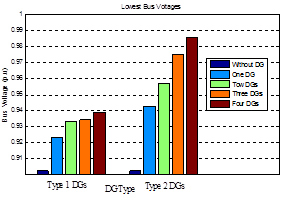Figure 2. A graph of the lowest bus voltages for different DG types and DG numbers.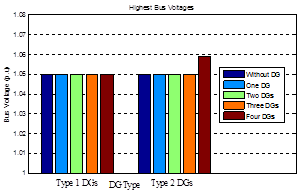Figure 3. A graph of the highest bus voltages for different DG types and DG numbers.

From fig. 2 above it can be seen that all the two cases resulted to an increase in the lowest bus voltage level. It also important to note that there was an increase for each additional DG added in the network up to the fourth DG. Note that the minimum voltage level for the base case is about 0.902 p.u recorded at bus 24. Fig. 3 shows the highest bus voltages for different DG types and DG numbers.

After installation of type 1 DG, there are still buses which are lower than the pre-specified voltage limit of 0.95 p.u. After installation of type 2 DG, the voltage levels of these buses are improved with minimum 0.943 p.u of bus number 25 with one DG. Since the most ideal case was to have this voltage as close to 1 p.u as possible it can be concluded that type 2 DG performed better in this case compared to type 1 DG. This is because its bus voltage levels with three DGs in the system are within the range of ±5%.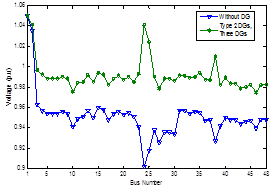Figure 4. Voltage profile before and after DG injection having optimum value.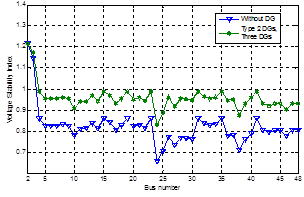Figure 5. Voltage Stability Index (SI) before and after DG installation at each bus of system.

Fig.4. shows voltage profiles improvement at various nodes for 48-bus Belin distribution system before and after connecting DG. Improvement in voltage stability was observed from Fig.5. In this figure the voltage stability index at each buses of system is shown

7. Conclusion

The solution methodology for optimal siting and sizing of distributed generation in Belin distribution system, considering precise models for distributed generations is presented in this paper. The benefits and consequences of distributed sources for improvement in voltage profile, voltage stability index and reduction on total network power loss have been analyzed in detail. According to the objective function, the best location in 48-bus Belin distribution system is in the order 24, 25 and 38 corresponding type 2 optimal DG sizes of MW and MVAR are 12.9831 + j5.9928, 10.0045 + j5.9844 and 4.9556 + j2.6225. The results of the proposed system depict that the optimal size of type 2 DG with three DGs is the maximum possible penetration levels of distributed generation in Belin distribution system in terms of improved voltage profile and reduced total real and reactive power loss of 0.915 MW and 7.71 MVAR.

Acknowledgements

The author would like to express grateful thanks to her supervisor Dr. Pyone Lai Swe, Associate Professor, Department of Electrical Power Engineering at Mandalay Technological University for her valuable suggestions and supervision through this research work. She also wants to thank all her teachers from Department of Electrical Power Engineering at Mandalay Technological University. The author offers her appreciation to her parents and brothers for their supports, encouragement and valuable guidance.

Appendix

Table 4. Data for 48-Bus Belin Distribution System.

 Sending Bus Receiving Bus R (p.u) X (p.u) Load at Receiving Bus P (MW) Q (MVAR) 1 2 0.00268 0.02232 0 0 2 3 0.00000 0.1418 0 0 3 4 0.01470 0.0356 0 0 3 15 0.02900 0.0818 0 0 3 19 0.02900 0.0818 0 0 3 23 0.02900 0.0818 0 0 3 31 0.03790 0.0920 0 0 3 35 0.03790 0.0920 0 0 3 41 0.08820 0.2486 0 0 4 5 0.36130 0.6974 0.45 0.24 4 6 0.30110 0.5812 0.61 0.32 4 7 0.37310 0.7199 0.44 0.23 4 8 0.07530 0.1453 1.14 0.60 4 9 0.15060 0.2906 1.1 0.58 4 10 0.16730 0.3228 4.5 2.37 4 11 0.18790 0.3626 2 1.05 4 12 0.17700 0.3416 1.8 0.948 4 13 0.00840 0.0161 0.15 0.079 4 14 0.08360 0.1614 3.94 2.075 15 16 0.09980 0.1294 0.925 0.487 15 17 0.30660 0.3975 2.204 1.158 15 18 0.23530 0.3051 1.429 0.753 19 20 0.03320 0.0719 4.52 2.38 19 21 0.13140 0.2844 0.375 0.197 19 22 0.06570 0.1153 4.146 2.185 23 24 0.30820 0.7736 4.846 2.554 23 25 0.25780 0.4522 4.279 2.254 23 26 0.02900 0.0559 4.4 2.317 23 27 0.13250 0.2868 4.85 2.554 23 28 0.09840 0.2130 2.042 1.074 23 29 0.05910 0.1278 3.946 2.08 23 30 0.08860 0.1917 3.42 1.8 31 32 0.00840 0.0161 0.015 0.0079 31 33 0.04180 0.0807 3.129 1.648 31 34 0.01250 0.0242 3.713 1.954 35 36 0.18720 0.2426 2.308 1.216 35 37 0.43680 0.5662 0.868 0.457 35 38 0.49930 0.6472 3.049 1.606 35 39 0.49930 0.6472 1.422 0.748 35 40 0.24970 0.3236 1.051 0.553 41 42 0.13380 0.2583 0.065 0.034 41 43 0.11710 0.2260 1.46 0.769 41 44 0.05850 0.1130 1.925 1.016 41 45 0.07190 0.1388 0.58 0.305 41 46 0.17560 0.3390 2.21 1.164 41 47 0.10040 0.1937 0.102 0.054 41 48 0.07780 0.1501 0.12 0.063

References

1. Satish Kansal, B.B.R. Sai, Barjeev Tyagi, Vishal Kumar "Optimal placement of distributed generation in distribution networks", International Journal of Engineering, Science and Technology,Vol. 3, No. 3, 2011, pp. 47-55.
2. Ackermann, T., Anderson, G. and Soder, L., 2001. "Distributed Generation: A Definition", Electric Power System Research, 57(3): 195-204.
3. Soroudi. A and M.Ehsan. "Multi objective distributed generation planning in liberized electricity market", in IEEE Proc. 2008, PP.1-7.
4. Borges, C.L.T. and Falcao, D.M., 2006. "Optimal Distributed Generation Allocation for Reliability, Losses and Voltage Improvement." Electric Power and Energy System, 28: 413-420.
5. Kim, T.E., 2001a. "A method for determining the introduction limit of distributed generation system in distribution system." IEEE Trans. Power Delivery, 4(2): 100-117.
6. Kim, T.E., 2001b. "Voltage regulation coordination of distributed generation system in distribution system." IEEE Trans. Power Delivery, 6(3): 1100-1117.
7. Tautiva, C. and Cadena, A., 2008. "Optimal Placement of Distributed Generation on Distribution Network." Proceeding of Transmission and Distribution Conference and Exposition-IEEE/PES-Bogota.
8. Chakravorty M, Das D. Voltage stability analysis of radial distribution networks. Int J Electr Power & Energy Syst 2001;23:129–35.
9. C. L. Wadhwa, ―Electrical Power system, New Age International Publication, 2010, Sixth Edition.

 Contents 1. 2. 3. 4. 5. 6. 6.1. 6.2. 7.
Article ToolsAbstractPDF(255K)